# Factoring with FOIL, Graphing Parabolas and Solving Quadratics Chapter Exam

Exam Instructions:

Choose your answers to the questions and click 'Next' to see the next set of questions. You can skip questions if you would like and come back to them later with the yellow "Go To First Skipped Question" button. When you have completed the practice exam, a green submit button will appear. Click it to see your results. Good luck!

### Page 1

#### Question 1 1. Expressing the equation below in the vertex form will give a value of 'h' = _____ .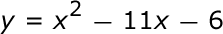#### Question 4 4. Solve the equation below.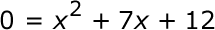### Page 2

#### Question 9 9. What is the b-value of the quadratic equation below?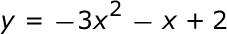### Page 3

#### Question 11 11. Which of the following are the solutions to the equation below?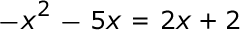#### Question 14 14. When completing the square on the quadratic equation listed below, how is c calculated?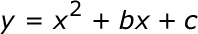#### Question 15 15. What would the first step be in completing the square of the following equation?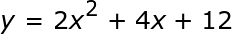### Page 4

#### Question 18 18. Rewrite the quadratic below in standard form: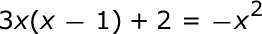#### Question 19 19. Find the value for c that needs to be added to both sides and rewrite the equation in the vertex form.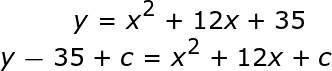### Page 6

#### Factoring with FOIL, Graphing Parabolas and Solving Quadratics Chapter Exam Instructions

Choose your answers to the questions and click 'Next' to see the next set of questions. You can skip questions if you would like and come back to them later with the yellow "Go To First Skipped Question" button. When you have completed the practice exam, a green submit button will appear. Click it to see your results. Good luck!

Support